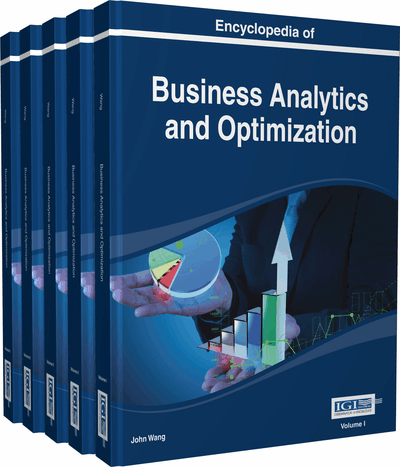# Isac's Cones in General Vector Spaces

Vasile Postolică (Romanian Academy of Scientists,“Vasile Alecsandri” University of Bacău, Faculty of Sciences, Bacău, România)
DOI: 10.4018/978-1-4666-5202-6.ch121

## Key Terms in this Chapter

Topology: Every collection of sets containing the empty and the environment space, closed to the infinite unions and the finite inclusions.

Seminorm: Any positive real function defined on a real or complex vector space, satisfying the absolute homogeneity property and the triangle inequality.

Isac’s (Nuclear or Supernormal) Cone: Each convex cone in any Hausdorff locally convex space with every seminorm majorized by at least a linear and continuous functional on it.

Hausdorff Locally Convex Space: Any topological vector space endowed with the topology generated by a family of seminorms, with any two different points separated by two disjoint neighborhoods.

Minkowski Functional: Every function associated to any non-emty subset of a vector space and defined by for each ).

Choquet Boundary: The boundary of any non-empty and compact subset in every Hausdorff locally convex space, with respect to an appropriate convex cone of real functions defined on it.

Convex Cone: Every non-empty subset of a vector space closed with respect to its addition and multiplication by positive scalars operations.

Efficiency: The quality of a point in a non-void set of an ordered vector space to be of minimum (maximum) in relation with a convex cone or to its appropriate translations.

Topological Vector Space: Each vector space with its basis definition operations continuous.

## Complete Chapter List

Search this Book:
Reset